# alternating current

(redirected from Alternating Currents)
Also found in: Dictionary, Thesaurus, Medical, Wikipedia.

## alternating current,

abbr. AC, a flow of electric charge that undergoes periodic reverses in direction. In North America ordinary household current alternates at a frequency of 60 times per second. See electricityelectricity,
class of phenomena arising from the existence of charge. The basic unit of charge is that on the proton or electron—the proton's charge is designated as positive while the electron's is negative.
; generatorgenerator,
in electricity, machine used to change mechanical energy into electrical energy. It operates on the principle of electromagnetic induction, discovered (1831) by Michael Faraday.
.

## Alternating Current

broadly defined, an electric current that changes with time. In engineering, an alternating current is understood to mean a periodic current. For such a current, the average values of the current and the voltage over a period are equal to zero. A period T is defined as the smallest time interval, measured in sec, that separates recurring changes of current and voltage (see Figure 1). Frequency f—the number of periods per sec, or 1/T—is an important characteristic of alternating currents.Figure 1. Graph of a periodic alternating current, i(t)

In the electric power systems of the USSR and most countries of the world, the established standard frequency is f = 50 hertz (Hz); in the USA it is 60 Hz. In communications engineering, high-frequency alternating currents are often used, with frequencies ranging from 100 kHz to 30 GHz. Alternating currents of widely differing frequencies, as well as pulsed currents, are used for special purposes in industry, medicine, and other areas of science and engineering.

AC systems are preferred for the transmission and distribution of electric power because the transformation of alternating voltages is quite simple and almost entirely free of power losses. Three-phase AC systems are widely used. A comparison of AC generators and motors and the corresponding DC machines shows that AC machines are smaller, simpler, more reliable, and less expensive for the same power rating. An alternating current can be rectified, for instance, by semiconductor rectifiers, and then converted by semiconductor inverters to an alternating current of a different controlled frequency. This permits the use of simple and inexpensive asynchronous and synchronous AC motors without commutators in various electric drives that require a continuous range of speeds.

Alternating current is widely used in communication systems, for example, radio, television, and long-distance wire telephony.

An alternating current is generated by an alternating voltage. An alternating electromagnetic field is created in the space surrounding a current-carrying conductor. This field causes energy oscillations in the AC circuit. This energy is periodically either stored in the magnetic or electric field or is returned to the electric power source. These energy oscillations cause reactive currents to flow through the AC circuit, causing an unproductive loading of the conductors and power source and thus leading to additional power losses. This fact is a drawback of power transmission in AC networks.

The magnitude of an alternating current is basically defined by a comparison of the average thermal effect of the alternating current with the thermal effect of a direct current of corresponding magnitude. The value of the current I thus obtained is called the effective value. Mathematically, this is the root-mean-square value of the current during one period. The effective value of the alternating voltage U is similarly defined. The effective values of voltage and current are the quantities measured by AC voltmeters and ammeters.

In the case that is both the simplest and the most important for practical applications, the instantaneous value i of the alternating current changes in time according to the sine law: i = Im sin (ωt + α),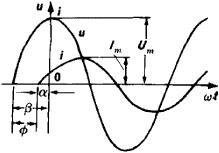Figure 2. Graph of voltage u and current i in an AC circuit with phase shift ϕ

where Im is the amplitude of the current, ω = 2πf is the angular frequency of the current, and α is the phase at t = 0. A sinusoidal (harmonic) current is created by a sinusoidal voltage of the same frequency; that is, u = Um sin (ωt + β), where Um is the amplitude of the voltage and β is the initial phase(see Figure 2). The effective values of the current and the voltage for alternating current are, respectively,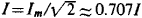and. For sinusoidal currents that satisfy the conditions for being quasi-stationary (the only currents considered in the following discussion), Ohm’s law is valid. (Ohm’s law in its differential form is also valid for nonquasi-stationary currents in linear circuits.) In the general case, the presence of inductance and/or capacitance in an AC circuit causes a phase shift ϕ = β – α. This shift is determined by the parameters of the circuit—the resistance r, the inductance L, and the capacitance C—and by the angular frequency ω. As a result of the phase shift, the average power P of the alternating current measured by a wattmeter is smaller than the product of the effective values of current and voltage: P = IU cos ϕ.Figure 3. Schematic diagram and graph of voltage u and current i in a circuit containing only the effective resistance r

In a circuit without inductance or capacitance, the current is in phase with the voltage (see Figure 3). In such a circuit, Ohm’s law for the effective values has the same form as Ohm’s law for DC circuits: I = U/r. Here r is the resistance of the circuit, determined by the actual power P consumed in the circuit: r = P/I2.

If an inductance L is present in the circuit, the alternating current will induce in L an electromotive force (emf) of self-induction eL = – L · di/dt = –ωLIM cos (ωt + α) = (ωLIm sin (ωt + α – π/2). The self-induced emf opposes changes of the current; in a circuit that contains only an inductance, the current will lag in phase behind the voltage by one-quarter of a period; that is, ϕ = π/2 (see Figure 4). The effective value of eL is equal to EL = IωL = IxL, where XL = ωL is the inductive reactance of the circuit. Ohm’s law for such a circuit has the form I = U/xL = U/ωL.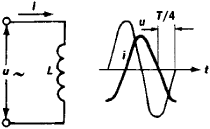Figure 4. Schematic diagram and graph of voltage u and current i in a circuit containing only the inductance L

When a capacitance C is connected to a voltage u, its charge is equal to q = Cu. Periodic changes in the voltage cause periodic changes in the charge and give rise to a capacitance current i = dq/dt = C· du/dt = ωCUm cos (ωt + β) = ωCUm sin (ωt + β + π/2). Thus, the sinusoidal alternating current that flows through the capacitance leads the voltage at the capacitance terminals by one-quarter of a period; that is, ϕ = – π/2 (see Figure 5). The effective values in such a circuit satisfy the relationship I = ωCU = U/xc, where xc = 1/ωC is the capacitive reactance of the circuit.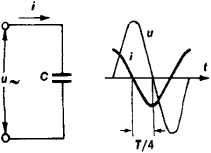Figure 5. Schematic diagram and graph of voltage u and current i in a circuit containing only the capacitance C

If an AC circuit consists of r, L, and C connected in series, its total impedance is, where x = xL – xC = ωL – 1/ωC is the reactance of the circuit. Correspondingly, Ohm’s law assumes the form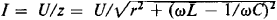, and the phase shift between the voltage and the current is determined by the ratio of the reactance of the circuit to its resistance: tan ϕ = x/r. In such a circuit the frequency ω of the forced oscillations generated by the source of alternating current may coincide with the resonant frequency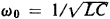. In this case, the inductive reactance and the capacitive reactance are equal (ωL = 1/ωC) and fully balance each other out, the magnitude of the current attains a maximum value, and the phenomenon of resonance occurs. Under conditions of resonance, the voltage across the inductance and the capacitance can significantly exceed the voltage at the circuit terminals and can frequently be many times as great.

Computations of sinusoidal AC circuits are facilitated by the construction of vector diagrams. It is customary to designate the vectors of sinusoidal currents and voltages by a point placed above the letter (İ, U̇). The lengths of the vectors are usually made equal to the effective values of I and U (on the scale of the diagram); the angles between the vectors are made equal to the phase shifts between the instantaneous values of the appropriate quantities. The algebraic addition of instantaneous values of any of the sinusoidal quantities then corresponds to the geometric addition of the vectors of these quantities.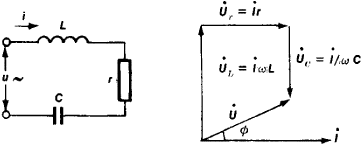Figure 6. Schematic and vector diagrams for an AC circuit with an inductance (L) and effective resistance (r) and a capacitance (C) connected in series

Figure 6 shows the vector diagram for an AC circuit with r, L, and C connected in series. The instantaneous value of the voltage across the terminals of this circuit is equal to the algebraic sum of the voltages across the resistance and across the reactance: u = uL + ur + uC. Therefore, U̇ = U̇L + U̇r + U̇C. In constructing the diagram, the current vector is taken as the reference vector, because for a nonbranching circuit the current is the same in all sections of the circuit. Inasmuch as the inductive voltage leads the current in phase by π/2 and the capacitive voltage lags behind the current by π/2, these voltages are in antiphase and will partially cancel each other in the series connection.

Vector diagrams visually illustrate the course of computations and serve as a control on the results of the computations. If requirements of scale are observed, such diagrams yield a graphical determination of the effective circuit voltage U and the phase-shift angle ϕ.

Calculations for branching circuits with quasi-stationary alternating currents are based on Kirchhoff’s laws. The method of complex quantities (a symbolic method) is usually used in these calculations. This method makes it possible to express in algebraic form the geometric operations performed with the AC vectors and thus to apply all the calculation methods for DC circuits to AC circuits.

In electric power systems, nonsinusoidal operation is usually undesirable, and special measures are taken to avoid such a mode of operation. However, nonsinusoidal conditions are inherent in the workings of electric communication circuits and semiconductor and electronic devices. If the average value of the current over a cycle is not equal to zero, the current must contain a constant component. To facilitate the analysis of circuits operating with nonsinusoidal currents, the current is represented as a sum of simple harmonic components. The frequencies of these harmonics are equal to integral multiples of the fundamental frequency: i = I0 + I1m sin (ωt + α1) + I2m sin (2ωt + α2) + ··· + Ikm sin (kωt + αk), where I0 is the constant component of the current, I1m sin (ωt + α1) is the first harmonic component (the fundamental), and all other terms are higher harmonics.

The computations used for nonsinusoidal currents in linear circuits are based on the superposition principle. Since xL and xC are functions of the frequency, these computations are conducted for each component. Algebraic addition of the results of such calculations yields the instantaneous value of current or voltage for the nonsinusoidal current.

### REFERENCES

Teoreticheskie osnovy elektrotekhniki, 3rd ed., part 2. Moscow, 1970
Neiman, L. R., and K. S. Demirchan. Teoreticheskie osnovy elektrotekhniki, vols. 1–2. Moscow-Leningrad, 1966.
Kasatkin A. S. Elektrotekhnika, 3rd ed. Moscow, 1974.
Polivanov K. M. Lineinye elektricheskie tsepi s sosredotochennymi postoiannymi. Moscow, 1972. (Teoreticheskie osnovy elektrotekhniki, vol.1.)

A. S. KASATKIN

## alternating current

[¦ȯl·tər‚nād·iŋ ¦kər·ənt]
(electricity)
Electric current that reverses direction periodically, usually many times per second. Abbreviated ac.

## Alternating current

Electric current that reverses direction periodically, usually many times per second. Electrical energy is ordinarily generated by a public or a private utility organization and provided to a customer, whether industrial or domestic, as alternating current.

One complete period, with current flow first in one direction and then in the other, is called a cycle, and 60 cycles per second (60 hertz) is the customary frequency of alternation in the United States and in all of North America. In Europe and in many other parts of the world, 50 Hz is the standard frequency. On aircraft a higher frequency, often 400 Hz, is used to make possible lighter electrical machines.

When the term alternating current is used as an adjective, it is commonly abbreviated to ac, as in ac motor. Similarly, direct current as an adjective is abbreviated dc.

The voltage of an alternating current can be changed by a transformer. This simple, inexpensive, static device permits generation of electric power at moderate voltage, efficient transmission for many miles at high voltage, and distribution and consumption at a conveniently low voltage. With direct (unidirectional) current it is not possible to use a transformer to change voltage. On a few power lines, electric energy is transmitted for great distances as direct current, but the electric energy is generated as alternating current, transformed to a high voltage, then rectified to direct current and transmitted, then changed back to alternating current by an inverter, to be transformed down to a lower voltage for distribution and use.

In addition to permitting efficient transmission of energy, alternating current provides advantages in the design of generators and motors, and for some purposes gives better operating characteristics. Certain devices involving chokes and transformers could be operated only with difficulty, if at all, on direct current. Also, the operation of large switches (called circuit breakers) is facilitated because the instantaneous value of alternating current automatically becomes zero twice in each cycle and an opening circuit breaker need not interrupt the current but only prevent current from starting again after its instant of zero value.

Alternating current is shown diagrammatically in Fig. 1. In this diagram it is assumed that the current is alternating sinusoidally; that is, the current i is described by the equation below,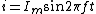where Im is the maximum instantaneous current, f is the frequency in cycles per second (hertz), and t is the time in seconds.

A sinusoidal form of current, or voltage, is usually approximated on practical power systems because the sinusoidal form results in less expensive construction and greater efficiency of operation of electric generators, transformers, motors, and other machines.

A useful measure of alternating current is found in the ability of the current to do work, and the amount of current is correspondingly defined as the square root of the average of the square of instantaneous current, the average being taken over an integer number of cycles. This value is known as the root-mean-square (rms) or effective current. It is measured in amperes. It is a useful measure for current of any frequency. The rms value of direct current is identical with its dc value. The rms value of sinusoidally alternating current is Im (see Fig. 1 and the equation). Other useful quantities are the phase difference &phiv; between voltage and current and the power factor.

The phase angle and power factor of voltage and current in a circuit that supplies a load are determined by the load. Thus a load of pure resistance, such as an electric heater, has unity power factor. An inductive load, such as an induction motor, has a power factor less than 1 and the current lags behind the applied voltage. A capacitive load, such as a bank of capacitors, also has a power factor less than 1, but the current leads the voltage, and the phase angle &phiv; is a negative angle.

Three-phase systems are commonly used for generation, transmission, and distribution of electric power. A customer may be supplied with three-phase power, particularly if a large amount of power is used or the use of three-phase loads is desired. Small domestic customers are usually supplied with single-phase power. A three-phase system is essentially the same as three ordinary single-phase systems, with the three voltages of the three single-phase systems out of phase with each other by one-third of a cycle (120 degrees), as shown in Fig. 2. The three-phase system is balanced if the maximum voltage in each of the three phases is equal, and if the three phase angles are equal, &frac13; cycle each as shown. It is only necessary to have three wires for a three-phase system (a, b, and c of Fig. 3) plus a fourth wire n to serve as a common return or neutral conductor. On some systems the earth is used as the common or neutral conductor.

Each phase of a three-phase system carries current and conveys power and energy. If the three loads on the three phases of the three-phase system are equal and the voltages are balanced, then the currents are balanced also. The sum of the three currents is then zero at every instant. This means that current in the common conductor (n of Fig. 3) is always zero, and that the conductor could theoretically be omitted entirely. In practice, the three currents are not usually exactly balanced, and either of two situations obtains. Either the common neutral wire n is used, in which case it carries little current (and may be of high resistance compared to the other three line wires), or else the common neutral wire n is not used, only three line wires being installed, and the three phase currents are thereby forced to add to zero even though this requirement results in some imbalance of phase voltages at the load.

The total instantaneous power from generator to load is constant (does not vary with time) in a balanced, sinusoidal, three-phase system. This results in smoother operation and less vibration of motors and other ac devices. In addition, three-phase motors and generators are more economical than single-phase machines.

AC circuits are also used to convey information. An information circuit, such as telephone, radio, or control, employs varying voltage, current, waveform, frequency, and phase. Efficiency is often low, the chief requirement being to convey accurate information even though little of the transmitted power reaches the receiving end. For further consideration of the transmission of information See Radio, Telephone

An ideal power circuit should provide the customer with electric energy always available at unchanging voltage of constant waveform and frequency, the amount of current being determined by the customer's load. High efficiency is greatly desired. See Circuit (electricity), Joule's law, Ohm's law

## alternating current

An electric current that varies periodically in value and direction, first flowing in one direction in the circuit and then flowing in the opposite direction; each complete repetition is called a cycle, and the number of repetitions per second is called the frequency; usually expressed in Hertz (Hz).

## alternating current

a continuous electric current that periodically reverses direction, usually sinusoidally
Site: Follow: Share:
Open / Close